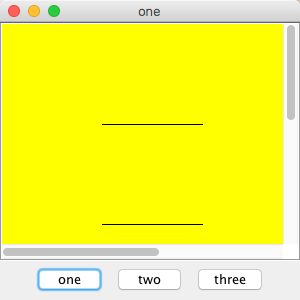# Drawing panel with scrolling - Musings

By: Nicholas Duchon.

Here's a (relatively) simple way to make a drawing scrollable.
Notice the use of JScrollPane in the code below```// File: cmsc330project1.java
// Author: Ted Malley - modifications by Nicholas Duchon
// Date: February 4, 2011
// Purpose: A simple GUI that shows various train tracks
// and trains, as well as switches, denoted by colored
// dots.
// > Also, add a panel of buttons to the bottom

import javax.swing.*;
import java.awt.*;

class Project1 {
public static void main(String[] args) {
ProjectWindow window = new ProjectWindow();
window.setVisible(true);
} // end main
} // end class Project1

class ProjectWindow extends JFrame {
public ProjectWindow() {
//Window characteristics
setTitle("one");
setDefaultCloseOperation(EXIT_ON_CLOSE);
setLocationRelativeTo(null); //centers the window
setSize(300, 300);

JPanel pb = new JPanel ();

Tracks a = new Tracks(100, 200, 200, 200);
Tracks b = new Tracks(100, 100, 200, 100);

// ND: put track and other stuff on graphics panel
//  see explanation below
GraphicsPanel gp = new GraphicsPanel ();
} // end ProjectWindow method
}
// end class ProjectWindow

// The following class is needed so the JScrollPane has a component to
// paint upon when scroll bar updates happen. Otherwise, the scroll panel will
// not update the drawing area. Other solutions involve setting up scroll bar
// listeners - this is much easier.
class GraphicsPanel extends JPanel {
public GraphicsPanel() {
setPreferredSize(new Dimension (500,500)); // works with scroll bars
setLayout(null);
setBackground(Color.yellow);
} // end constructor

public void paintComponent (Graphics g) {
super.paintComponent(g); // get background color to work
for (Component x: this.getComponents()) x.paint (g); // draw children
} // end method paintMe Graphics2D
} // end class GraphicsPanel

// class Tracks extends JPanel
class Tracks extends JComponent {
private double x1 = 0;
private double y1 = 0;
private double x2 = 0;
private double y2 = 0;

public Tracks(double x1, double y1, double x2, double y2) {
this.x1 = x1;
this.y1 = y1;
this.x2 = x2;
this.y2 = y2;
} // end double,double,double,double constructor

public void paint (Graphics g) {
super.paint(g);
Graphics2D g2d = (Graphics2D) g;
g.setColor (Color.black);
g.drawLine ((int)x1, (int)y2, (int)x2, (int)y2);
} // end paint
} // end class Track```

ND.
` `